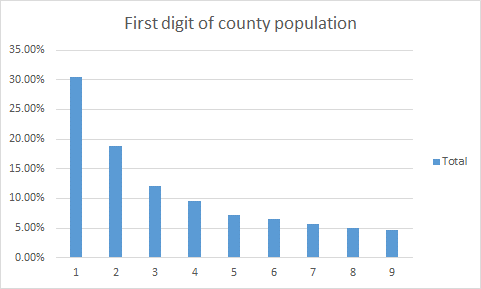# Benford’s law

Benford’s law is an observation about the leading digits of the numbers found in real-world data sets. Intuitively, one might expect that the leading digits of these numbers would be uniformly distributed so that each of the digits from 1 to 9 is equally likely to appear. In fact, it is often the case that 1 occurs more frequently than 2, 2 more frequently than 3, and so on. This observation is a simplified version of Benford’s law. More precisely, the law gives a prediction of the frequency of leading digits using base-10 logarithms that predicts specific frequencies which decrease as the digits increase from 1 to 9.

It is used often in Applications to Fraud Detection

It is difficult for humans to manually construct distributions that satisfy Benford’s law. Fraudulent numerical data can often be identified by simply looking at the frequency of first digits, although often in practice more than one digit is used for a more precise check. In particular, Benford’s law has been applied to entries on tax forms, election results, economic numbers, and accounting figures.

This phenomenon occurs generally in many different instances of real-world data. It becomes more pronounced and more likely when more data is combined together from different sources. Not every data set satisfies Benford’s law, and it is surprisingly difficult to explain the law’s occurrence in the data sets it does describe, but nevertheless it does occur consistently in well-understood circumstances. Scientists have even begun to use versions of the law to detect potential fraud in published data (tax returns, election results) that are expected to satisfy the law.

Here is a histogram of the areas of 196 196 196 countries (data taken from Wikipedia). The units are km2 \text{km}^2 km2.

Here is a table with percentages. The “BL prediction” column is the percentage that Benford’s law predicts for each digit. (These numbers will be explained in the full statement of the law in the next section.)

Here is a histogram of the population of each of the 3,142 counties or county equivalents in the United States (data taken from Wikipedia).

Here is a table with percentages.

So Benford’s law appears to predict the data in both examples quite well.

Notes:

I’m not going to explain here how the law work.

However when using big number this is a marvelous way to create application for checking anomalies in a set of data .

Not well advertised as tool and ditto here it is!

This site uses Akismet to reduce spam. Learn how your comment data is processed.